# Data Jobs: What does a Data Analyst Do?

Data Analysts get a bad wrap. With the advent of the Data Scientist, Data Analysts are often viewed as Data Scientists lite, however I feel that is not the honest case. Truth is, there is a lot of overlap between the two fields. I will dive deeper into what a Data Scientist is in a future article, but just know my opinion is the definition of Data Scientist as a job is still a bit fuzzy and I think the job title may eventually be broken into a few different titles to better define the differences.

## Data Analyst

So what does a Data Analyst do?

A lot actually. You could put 10 data analysts into a room and you would get ten different answers to this question. So the best I can do here is make sweeping generalities. As the old saying goes “Your results may vary”

In general, data analysts perform statistical analysis, create reporting, run ad-hoc queries from data warehouses, create data visualizations, create and maintain dashboards, perform data mining, and create machine learning models (yes, ML is not only for data scientists). Their assignments are business driven. A data analysts is either embedded with a business unit (financial planning, fraud, risk management, cyber security, etc.) or working in a centralized reporting/analytics team. They use their skills to provide reporting and analytics for the business.

## Tools used by Data Analysts

• SQL – MySql, SQL Server, Oracle, Teradata, Postgres – whether simply querying a data warehouse or creating and managing a local data mart, data analysts need to be advanced SQL programmers
• Visualization tools – Tableau, Qlik, Power BI, Excel, analysts use these tools to create visualizations and dashboards
• Python/R – Data analysts should be familiar with languages like Python or R to help manage data and perform statistical analysis or build machine learning models
• Spreadsheets – Excel, Google Sheets, Smart Sheets are used to create reports, and pivot tables used to analyze the data
• ETL tools – SSIS, Alteryx, Talend, Knime, these tools are design to move data to and from databases, CSV files, and spreadsheets. Until the data is in a usable format, analysis cannot be performed.

## Educational Requirements

Typically a data analyst position will ask for a bachelors degrees, preferably in computer science, statistics, database management or even business. While the barrier to entry for a data analyst job is generally not as high as a data scientist, that does not mean you cannot make a meaningful and well paid career as a data analyst. Also, the demand for data professionals seems to keep going up and up and it most likely will for the foreseeable future.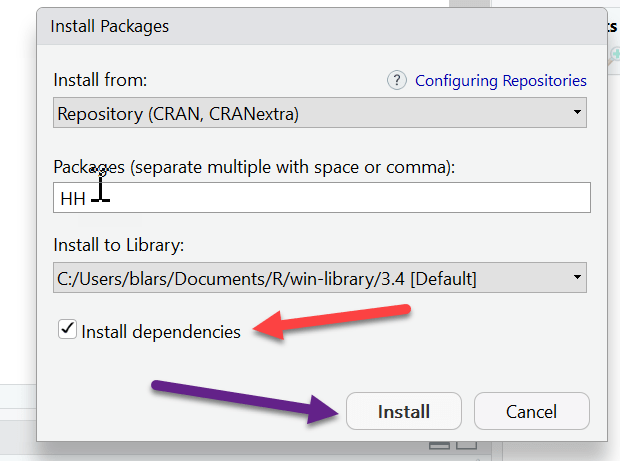# R: Installing Packages with Dependencies

Usually installing packages in R is as simple as

`install.packages("package name")`

However sometimes you will run into errors. This could be due to the fact that the package you are trying to install has what is known as a dependency. What this means is that in order for the package to properly install and run, it requires another package to  already be installed.

You can think of this like trying to install an add-on for Excel like PowerQuery without having Excel installed in the first place. Clearly it would not work.

Now if you are lucky enough to know exactly what package(s) needs to be installed first, then you can simply install it and be on your way. However, most of the time we are not that lucky. And in the case of some packages, you may need to install up to a dozen packages up front to get it to work.

`install.packages("package name", dependencies = TRUE)`

Remember in R, Boolean (TRUE and FALSE) must be all capital letters or R will not recognize them as Boolean.

If you are using RStudio, you can install the package using the GUI

At the top, got to Tools and select Install Packages from the drop down.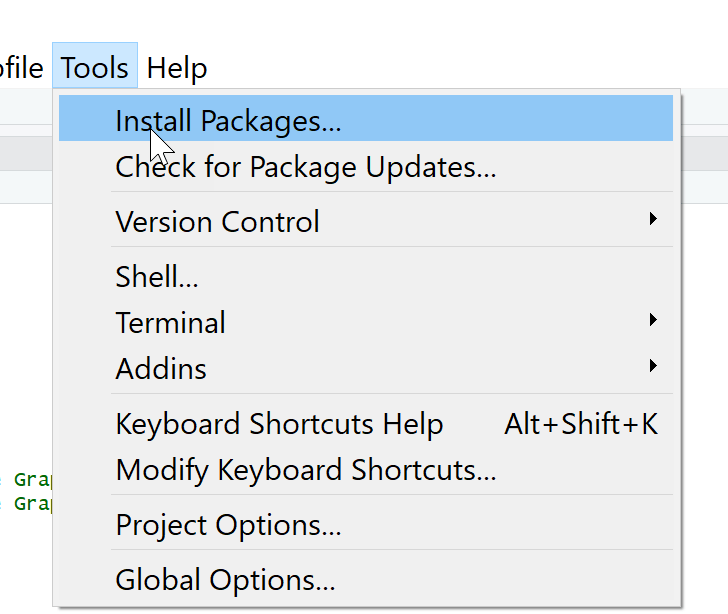Start typing the package you want in the box, it will pop up in the windowFinally, make sure install dependencies and checked and click install.# R: Manually entering data

You can use the data frame edit() function to manually enter / edit data in R.

Start by creating a data frame.

Note I am initializing each of the columns to datatype(0). This tells R that I while I want the name column to be a character and the age column to be numeric, I am leaving the size dynamic. You can set size limits during the initialization phase if you so choose.

` dfe <- data.frame(name=character(0), age = numeric(0), jobTitle = character(0))`

Now, let’s use the edit() function to add some data to our data frame

`dfe<- edit(dfe)`

When the new window pops up, fill in the data and simply click the X when you are doneYou may get warning messages when you close out your edit window. These particular messages I got simply informed me that name and jobTitle were set as factors by R. Remember in R, warnings just want you to be aware of something, they are not errors.

Now if you run dfe, you can see your data frame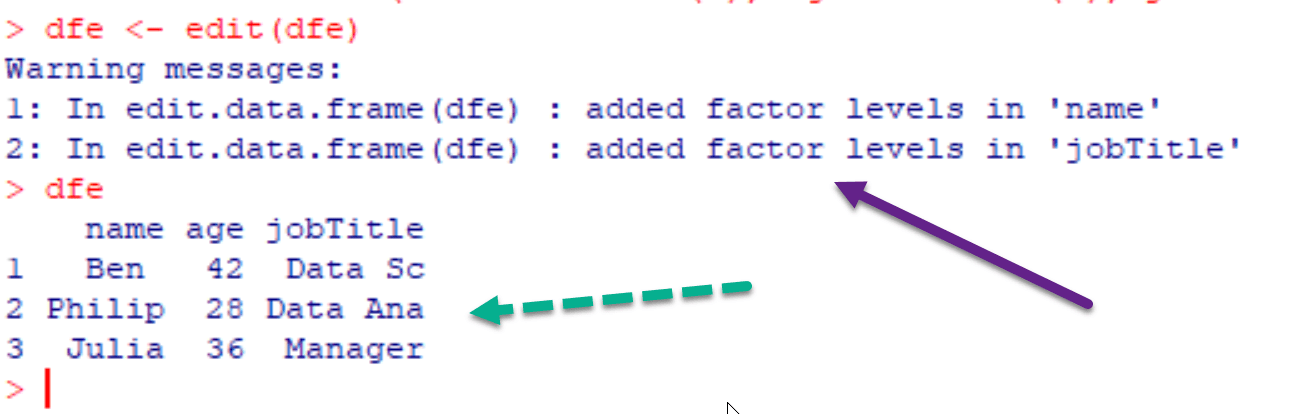By running the edit() function again, you can edit the values that currently exist in the data frame.  In this example I am going to change Philip’s age from 28 to 29

If you want to add a column to the data frame, just add data to the next empty column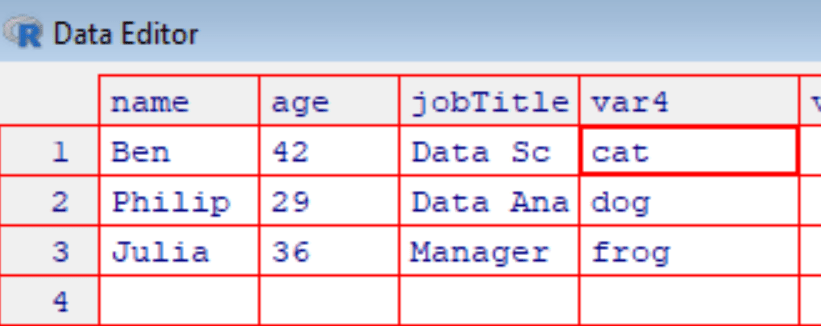You can just close out now and rename the column in R, or just click on the column header and you will be able to rename it there.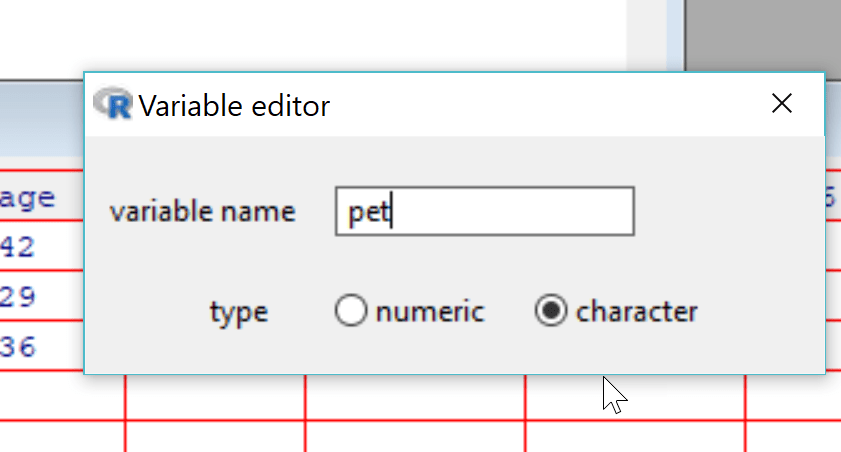Now we have a new column listing pets

```  name   age jobTitle pet
1 Ben    42  Data Sc  cat
2 Philip 29  Data Ana dog
3 Julia  36  Manager  frog```

You can use the edit() function to manually edit existing data sets or data imported from other sources.

Below, I am editing the ChickWeight data set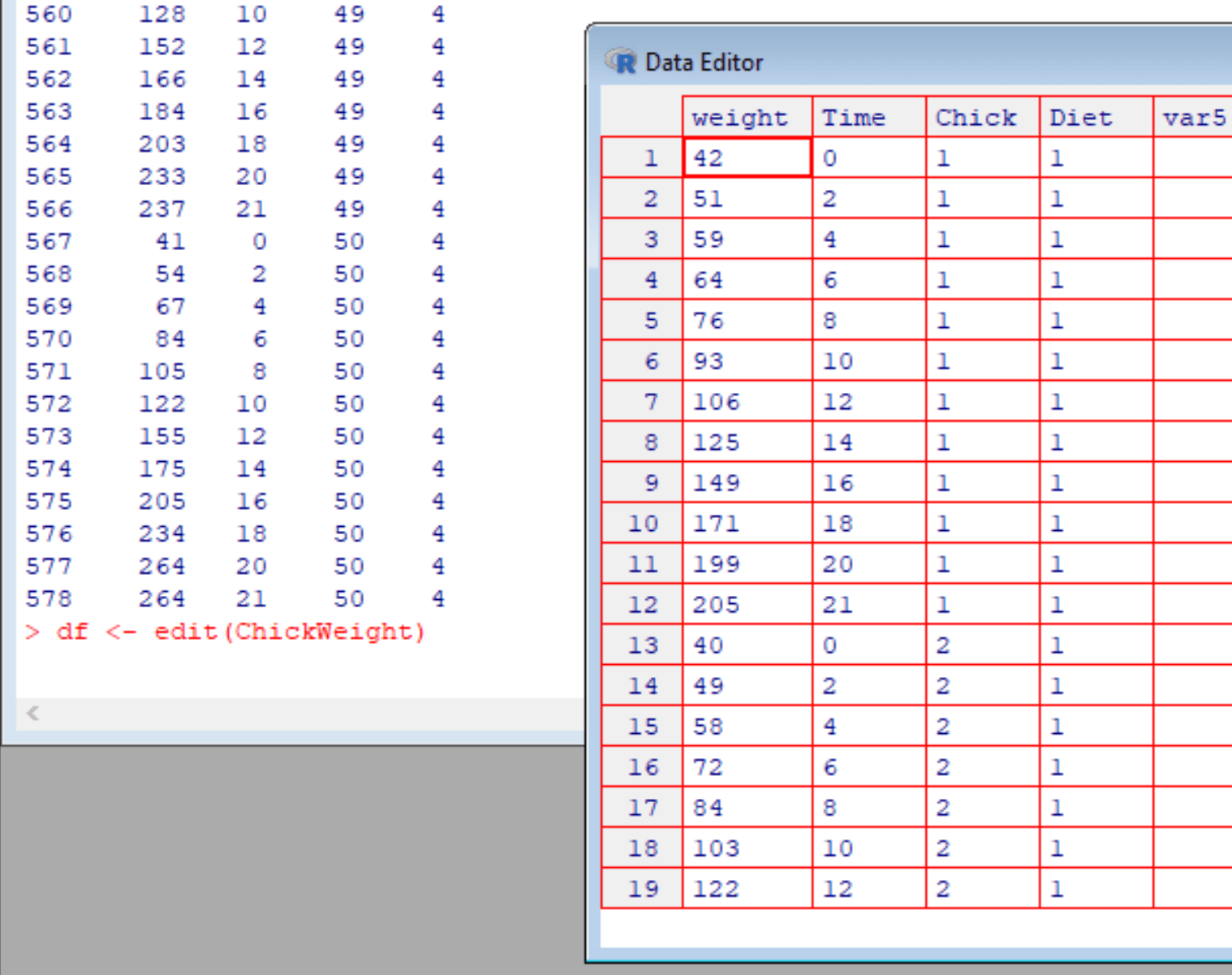# R: Connecting to SQL Server Database

You can query data from a SQL Server database directly from R using the RODBC package.

`install.packages("RODBC")`

First you need to form a connection

```library(RODBC)
##connection string
cn <- odbcDriverConnect(connection="Driver={SQL Server Native Client 11.0};server=localhost; database=SSRSTraining;trusted_connection=yes;")```

We use the odbcDriverConnect() function. Inside we pass a connection = value

Driver = {SQL Server Native Client 11.0};  — this is based on the version of SQL Server you have

server=localhost;  — I used localhost because the SQL Server was on the same computer I was working with. Otherwise, pass the server name

database=SSRSTraining; — name of database I want to work with

trusted_connection=yes; — this means I am able to pass my Windows credentials.

If you don’t have a trusted connect pass the user Id and password like this

Note each parameter is separated by a semicolon

### Query the database

```> ##passes query to SQL Server
> df <- sqlQuery(cn, "select * FROM [SSRSTraining].[dbo].[JobDataSet]")

Name              Job Hours Complete
1  Sally Predictive Model     1        n
2 Philip      Maintanence    10        n
3    Tom    Ad-hoc Report    12        y
4    Bob             SSRS     3        y
5 Philip         Tableau      7        n
6    Tom         Tableau      9        n```

using sqlQuery() – pass through the connection string (cn) and enclose your query in ” ”

# R: Importing Excel Files

To work with Excel files in R, you can use the readxl library

```> library(readxl)
>
# A tibble: 6 x 4
Name   Job              Hours Complete
<chr>  <chr>            <dbl> <chr>
1 Sally  Predictive Model    1. n
2 Philip Maintanence        10. n
3 Tom    Ad-hoc Report      12. y
4 Bob    SSRS                3. y
5 Philip Tableau             7. n
6 Tom    Tableau             9. n```

By default, read_excel() reads only the first sheet in the Excel file. To read other sheets using the sheet key word.

```> ## read  sheet 2
> df2 <- read_excel("r_excel.xlsx", sheet =2)

# A tibble: 6 x 2
Animal Num_Legs
<chr>     <dbl>
1 Dog          4.
2 Duck         2.
3 Snake        0.
4 Horse        4.
5 Spider       8.
6 Human        2.```

The next example, we are reading range B2 – C6 on sheet 1 (same as Excel’s range function)

```> ## read sheet 1, range B2 - C6
> df3 <- read_excel("r_excel.xlsx", sheet =1, range = "B2:C6")
> df3
# A tibble: 4 x 2
`Predictive Model`   `1`
<chr>              <dbl>
1 Maintanence          10.
3 SSRS                  3.
4 Tableau               7.```

In this last example, we are importing only the first 4 rows on sheet 2

```> ## read sheet 2, first 4 rows only
> df4 <- read_excel("r_excel.xlsx", sheet = 2, n_max = 4)
> df4
# A tibble: 4 x 2
Animal Num_Legs
<chr>     <dbl>
1 Dog          4.
2 Duck         2.
3 Snake        0.
4 Horse        4.```

# R Shiny: Introduction

Shiny is an R Studio application that allows you to how host your R code on a website. This is a great method for creating a dashboard or deploying a predictive model. The best part is, it is free. There are, however, paid services associated with R Studio and Shiny if you want to get professional support and such.

In this lesson, we are going to learn to build a Shiny server application step by step.

Let’s start at the beginning. First off, to use Shiny, you need to have R Studio installed on your computer. Once you have that, you will need to install the Shiny package

install.packages(‘shiny’)

Next, we are going to learn about the three parts of a Shiny server application.

Below is the basic framework of a Shiny app:

```library(shiny)

ui <- fluidPage("Test")
server <- function(input, output) {}
shinyApp(ui = ui, server = server)```

Let’s break this down:

ui: ui is the user interface. This is the code that makes up the web page the user will launch and interact with. Anyone familiar with HTML, this is the basic markup. This is where you set the wording, font, colors, what kind of object will be on the page, etc

server: server is the brains of the operation. This is where you load and manipulate any data being used in your page. Again, if you are a web developer, think Java Script here.

shinyApp: this is the code that launches your App.

If you run the code above, you will see something like this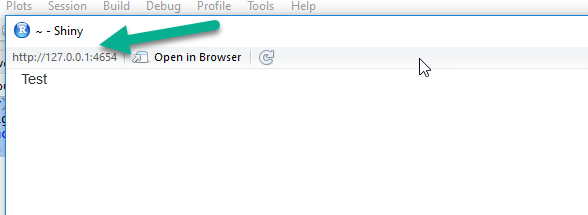Note the IP address in the upper left. If you copy this into a browser of your choice, it will run there too.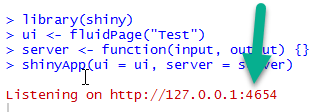You will see the same address in the R Console. As long as the R Shiny server is running, you will see this Listening command. For those not familiar, the address 127.0.0.1 is called a pingback address. This is the internal address of the NIC card on your machine. This means the server is running on the machine you are on. The :4654 is the port my computer grabbed. This can be different for you. The easiest way for me to explain the port without going too deep into network theory is: think of it like a TV channel. From your computer, you could technically be running hundreds of these R Shiny servers. The port (TV channel) is what tells the computer which one of these web pages you want to visit.

If you happen to be on a network, you can go to another computer and type in your host computers network IP address (you can get it by going to a cmd console and typing ipconfig). It will be the address I have the arrows pointing to in my exampleThen, using my example, type http://10.171.46.91:4654 into the second computer’s browser and you should see your Shiny website.

### Step 2

Let’s see if we can make our website more interesting, let’s start easy, we will format the text.

First close out your shiny window to end the session.

Change your ui line to match the one below.

ui <- fluidPage(h1(“Test”))

library(shiny)
ui <- fluidPage(h1(“Test”))
server <- function(input, output) {}
shinyApp(ui = ui, server = server)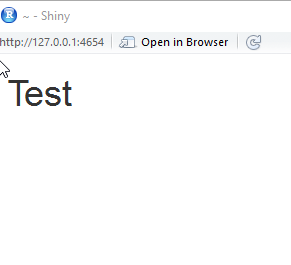### Step 3

Okay, let’s make it a little more interesting by adding some detail to our page. We will do so by using panel methods in our fluidPage()

```library(shiny)

ui <- fluidPage(
titlePanel("Title Panel"),
sidebarPanel("Sidebar Panel"),
mainPanel("Main Panel")
)
server <- function(input, output) {}
shinyApp(ui = ui, server = server)```

Notice your page is now segmented into a title bar, a sidebar and a main panel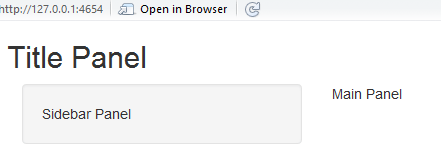Okay, now let’s actually add some R data magic to this.

```library(shiny)
library(datasets)
mpgData <- mtcars

ui <- fluidPage(
titlePanel("Mpg vs Cylinder Plot"),
sidebarPanel("Sidebar Panel"),
mainPanel(  plotOutput("mpgPlot"))
)
server <- function(input, output) {
output\$mpgPlot <- renderPlot({
boxplot(mpg~cyl, data= mpgData)})
}
shinyApp(ui = ui, server = server)```

Let’s break down the new code. First thing first, we are loading the built in datasets library and grabbing the mtcars data set from it.

library(datasets)

mpgData <- mtcars

Next we are going to skip down to the server section next as this is where we work with our data:

First thing you will notice is function(input, output) – this is a standard call that allows you to pass information back and forth through the server code. In our example, we are only using the output right now.

We create a variable called output\$mpgPlot to which we pass the shiny method renderPlot.  Inside that method, we are simply creating a simple boxplot comparing mpg to cyl using the mtcars data set that we labeled mpgData

```server <- function(input, output) {
output\$mpgPlot <- renderPlot({
boxplot(mpg~cyl, data= mpgData)})
}```

The boxplot we rendered as output\$mpgPlot is now passed to our ui

Next, in the ui I renamed the title panel and in the mainPanel, I added the command plotOutput(“mpgData”). That will allow me to post the boxplot I created in the server to the ui web page.

```ui <- fluidPage(
titlePanel("Mpg vs Cylinder Plot"),
sidebarPanel("Sidebar Panel"),
mainPanel(  plotOutput("mpgPlot"))
)```

If you run the code, it should look like this: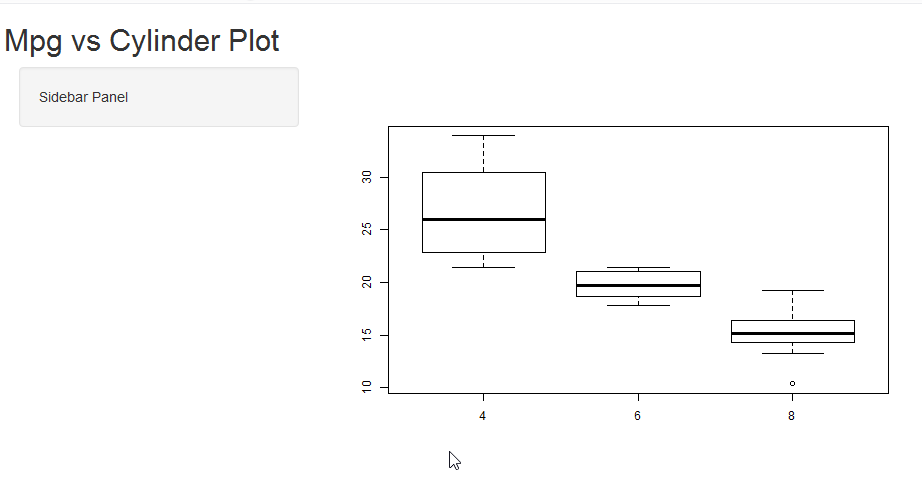### Step 4

Now let’s add a little interactivity to our web page. We are going to give the user the option to view the boxplots with or without the outliers taken into effect.

```library(shiny)
library(datasets)
mpgData <- mtcars

ui <- fluidPage(
titlePanel("Mpg vs Cylinder Plot"),
sidebarPanel(checkboxInput("outliers", "Show outliers", FALSE)),
mainPanel(
plotOutput("mpgPlot"))
)
server <- function(input, output) {
output\$mpgPlot <- renderPlot({
boxplot(mpg~cyl, data= mpgData,
outline = input\$outliers)})
}
shinyApp(ui = ui, server = server)```

This involves only two changes to our code

First we will add a checkbox to our sidebar, in the ui we will alter the sidebarPanel line to look like this

sidebarPanel(checkboxInput(“outliers”, “Show outliers”, FALSE)),

The breakdown of the syntax here is

checkboxinput(variable name, display text, default )– our default is set to FALSE so our box will be unchecked by default

Next, we will alter our boxplot to take into account the checkbox input

boxplot(mpg~cyl, data= mpgData,
outline = input\$outliers)

— notice the variable name is input\$outliers  It is a composite of the variable from our checkboxinput method and “input” from out function(input, output) function that opens up our server.

UncheckedChecked# R: Text Mining (Pre-processing)

This is part 2 of my Text Mining Lesson series. If you haven’t already, please check out part 1 that covers Term Document Matrix: R: Text Mining (Term Document Matrix)

Okay, now I promise to get to the fun stuff soon enough here, but I feel that in most tutorials I have seen online, the pre-processing of text is often glanced over. It was always (and often still is) a real sore spot for me when assumptions are made as to my knowledge level. If you are going to throw up a block of code, at least give a line or two explanation as to what the code is there for, don’t just assume I know.

I remember working my way through many tutorials where I was able to complete the task by simply copying the code, but I didn’t have a full grasp of what was happening in the middle. I this lesson, I am going to cover some of the more common text pre-processing steps used in the TM library. I am going to go into some level of detail and make some purposeful mistakes so hopefully when you are done here you will have a firm grasp on this very important step in the text mining process.

Let’s start by getting our libraries

```install.packages("tm") # if not already installed
install.packages("SnowballC")

library(tm)
library(SnowballC)```

Now, let’s load our data. For this lesson we are going to use a simple vector.

```wordVC <- c("I like dogs!", "A cat chased the dog.", "The dog ate a bone.",
"Cats make fun pets.", "The cat did what? Don't tell the dog.",
"A cat runs when it has too, but most dogs love running!")```

Now let’s put this data into a corpus for text processing.

```corpus <- (VectorSource(wordVC))
corpus <- Corpus(corpus)
summary(corpus)```

Here is the outputNow for a little frustration. Let’s say you want to see what is in text document 4. You could try

`inspect(corpus)`

But this will be your output, not really what you are looking for.If you want to see the actual text- try this instead

`corpus[]\$content`

Now you can see the textAs we go through the text pre-processing, we are going to use the following For Loop to examine our corpus

`for (i in 1:6) print (corpus[[i]]\$content)`

Output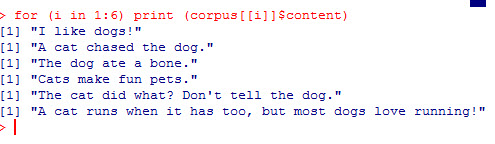### Punctuation

Punctuation generally adds no value to text mining when utilizing standard numeric based data mining algorithms like clustering or classification. So it behooves us to just remove it.

To do so, the tm package has a cool function called tm_map() that we can pass arguments to, such as removePunctuation

``````corpus <- tm_map(corpus, content_transformer(removePunctuation))

```for (i in 1:6) print (corpus[[i]]\$content)```

Note, you do not need the for loop, I am simply running it each time to show you the progress.

Notice all the punctuation is gone now.### Stopwords

Next we are going to get rid of what are known as stopwords. Stopwords are common words such as (the, an, and, him, her). These words are so commonly used that they provide little insight as to the actual meaning of the given text.  To get rid of them, we use the following code.

```corpus <- tm_map(corpus, content_transformer(removeWords),
stopwords("english"))
for (i in 1:6) print (corpus[[i]]\$content)```

If you look at line 2, “A cat chase  dog”, you will the word “the” has been removed. However, if you look at the next line down, you will notice “The” is still there.WHY?

Well it comes down to the fact that computers do not treat T and t as the same letter, even though they are. Capitalized letters are viewed by computers as separate entities. So “The” doesn’t match “the” found in the list of stopwords to remove.

For a full list of R stopwords, go to: https://github.com/arc12/Text-Mining-Weak-Signals/wiki/Standard-set-of-english-stopwords

So how do we fix this?

### tolower

Using tm_map with the “tolower” argument will make all the letters lowercase. If we then re-run our stopwords command, you will see all the “the” are gone

```corpus <- tm_map(corpus, content_transformer(tolower))
corpus <- tm_map(corpus, content_transformer(removeWords),
stopwords("english"))
for (i in 1:6) print (corpus[[i]]\$content)```

Output### stemming

Next we will stem our words. I covered this in the last lesson, but it bears repeating. What stemming does is attempt to remove variants of words. In our example, pay attention to the following words (dog, dogs, cat, cats, runs, running)

```corpus <- tm_map(corpus, stemDocument)
for (i in 1:6) print (corpus[[i]]\$content)```

Notice the words are now (dog, cat, run)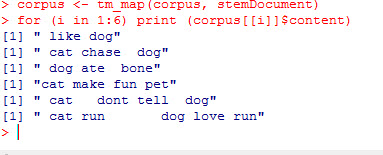### Whitespace

Finally, let’s get rid of all this extra white space we have now.

``````corpus <- tm_map(corpus, stripWhitespace)
for (i in 1:6) print (corpus[[i]]\$content)``````

Output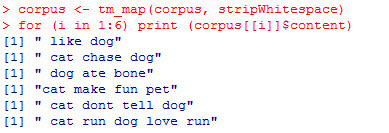### removeNumbers

I didn’t use this argument with my tm_map() function today because I did not have any numbers in my text. But if I, the command would be as follows

`corpus <- tm_map(corpus, content_transformer(removeNumbers))`

# R: Text Mining (Term Document Matrix)

There are a bounty of well known machine learning algorithms, both supervised (Decision Tree, K Nearest Neighbor, Logistical Regression) and unsupervised (clustering, anomaly detection). The only catch is that these algorithms are designed to work with numbers, not text. The act of using numeric based data mining methods on text is known as duo-mining.

So before you can utilize these algorithms, you first have to  transform text into a format suitable for use in these number based algorithms. One of the most popular methods people first learn is how to create a Term Document Matrix (TDM). The easiest way to understand this concept is, of course, to do an example.

```install.packages("tm") # if not already installed
library(tm)```

Now let’s create a simple vector of strings.

```wordVC <- c("I like dogs", "A cat chased the dog", "The dog ate a bone",
"Cats make fun pets")```

Now we are going to place the strings into a data type designed for text mining (from the tm package) called corpus. A corpus simply means the full collection of text you want to work with.

```corpus <- (VectorSource(wordVC))
corpus <- Corpus(corpus)
summary(corpus)```

Output:

``` Length Class Mode
1 2 PlainTextDocument list
2 2 PlainTextDocument list
3 2 PlainTextDocument list
4 2 PlainTextDocument list```

As you can see from the summary, the corpus classified each string in the vector as a PlaintextDocument.

Now let’s create our first Term Document Matrix

```TDM <- TermDocumentMatrix(corpus)
inspect(TDM)```

Output: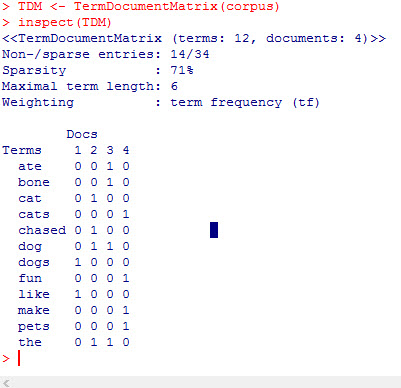As you can see, we now have a numeric representation of our text. Each row represents a word in our text and each column represents an individual sentence. So if you look at the word dog, you can see it appears once in sentence 2 and 3, (0110). While bone appears once in sentence 3 only (0010).

## Stemming

One immediate issue that jumps out at me is that R now sees the words cat & cats and dog & dogs as different words, when really cats and dogs are just the plural versions of cat and dog. Now there may be some more advanced applications of text mining that you would want to keep the two words separate, but in most basic text mining applications, you would want to only keep one version of the word.

Luckily for us, R makes that simple. Use the function tm_map with the argument stemDocument

`corpus2 <- tm_map(corpus, stemDocument)`

make a new TDM

```TDM2 <- TermDocumentMatrix(corpus2)
inspect(TDM2)```

Now you see only the singular of cat and dog exist in our list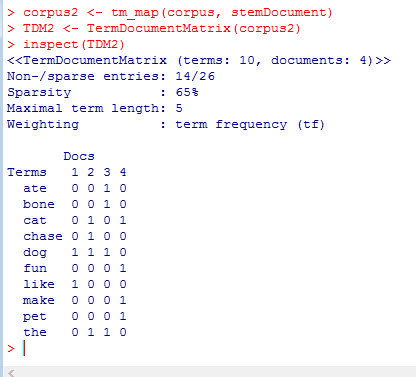If you would like, you can also work with the transpose of the TDM called the Document Term Matrix.

```dtm = t(TDM2)
inspect(dtm)```

dfsaI’ll get deeper into more pre-processing tasks, as well as ways to work with your TDM in future lessons. But for now, practice making TDMs see if you can think of ways that you can use TDMs and DTMs with some machine learning algorithms you might already know (decision trees, logistic regression).

# R: Creating a Word Cloud

Word Clouds are great visualization techniques for dealing with text analytics. The idea behind them is they display the most common words in a corpus of text. The more often a word is used, the larger and darker it is.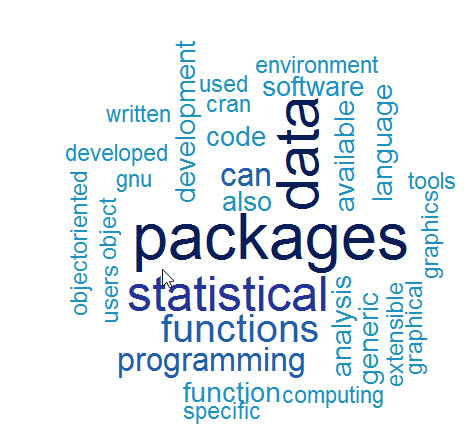Making a word cloud in R is relatively easy. The tm and wordcloud libraries from R’s CRAN repository is used to create one.

```library(tm)
library(wordcloud)

```

If you do not have either of these loaded on your machine, you will have to use the following commands

```install.packages("tm")
install.packages("wordcloud")```

Now in order to make a word cloud, you first need a collection of words. In our example I am going to use a text file I created from the Wikipedia page on R.

Now let’s load the data file.

```text <- readLines("rWiki.txt")
 "R is a programming language and software environment
 "The R language is widely used among statisticians and
 "Polls, surveys of data miners, and studies of scholarly
 "R is a GNU package. The source code for the R
 "General Public License, and pre-compiled binary versions
 "R is an implementation of the S programming language "
>```

Notice each line in the text file is an individual element in the vector –  text

Now we need to move the text into a tm element called a Corpus. First we need to convert the vector text into a VectorSource.

```wc <- VectorSource(text)
wc <- Corpus(wc)```

Now we need to pre-process the data. Let’s start by removing punctuation from the corpus.

`wc <- tm_map(wc, removePunctuation)`

Next we need to set all the letters to lower case. This is because R differentiates upper and lower case letters. So “Program” and “program” would treated as 2 different words. To change that, we set everything to lowercase.

`wc <- tm_map(wc, content_transformer(tolower))`

Next we will remove stopwords. Stopwords are commonly used words that provide no value to the evaluation of the text. Examples of stopwords are: the, a, an, and, if, or, not, with ….

`wc <- tm_map(wc, removeWords, stopwords("english"))`

Finally, let’s strip away the whitespace

`wc <- tm_map(wc, stripWhitespace)`

Now let us make our first word cloud

The syntax is as follows – wordcloud( words = corpus, scale = physical size, max.word = number of words in cloud)

`wordcloud(words = wc, scale=c(4,0.5), max.words=50)`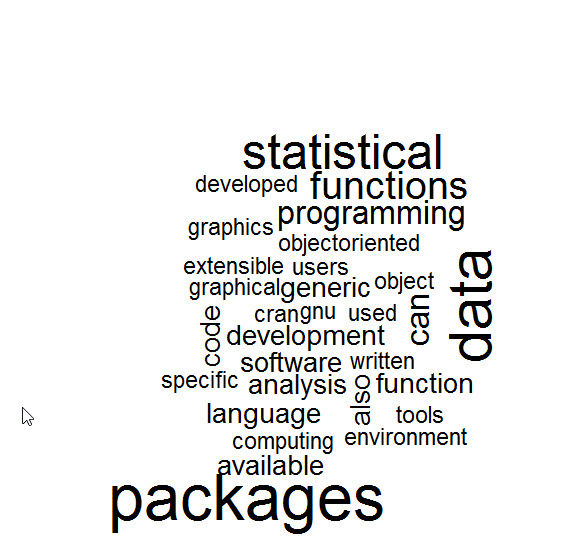Now we have a word cloud, let’s add some more elements to it.

random.order = False brings the most popular words to the center

`wordcloud(words = wc, scale=c(4,0.5), max.words=50,random.order=FALSE)`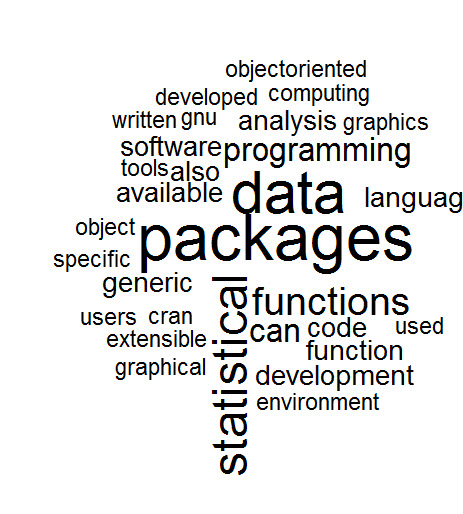To add a little more rotation to your word cloud use rot.per

```wordcloud(words = wc, scale=c(4,0.5), max.words=50,random.order=FALSE,
rot.per=0.25)```

Finally, lets add some color. We are going to use brewer.pal.  The syntax is brewer.pal(number of colors, color mix)

```cp <- brewer.pal(7,"YlOrRd")
wordcloud(words = wc, scale=c(4,0.5), max.words=50,random.order=FALSE,
rot.per=0.25, colors=cp)```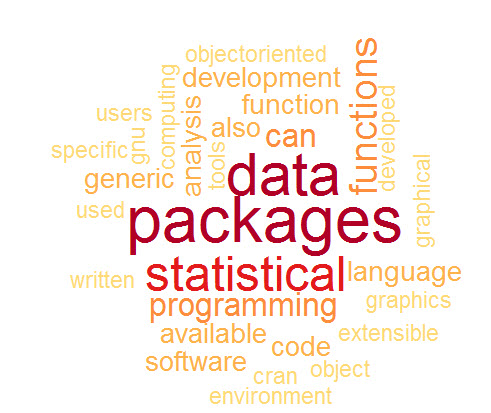# R: K-Means Clustering- Deciding how many clusters

In a previous lesson I showed you how to do a K-means cluster in R. You can visit that lesson here: R: K-Means Clustering.

Now in that lesson I choose 3 clusters. I did that because I was the one who made up the data, so I knew 3 clusters would work well. In the real world it doesn’t work that way. Choosing the right number of clusters is one of the trickier parts of performing a k-means cluster.

If you go over to Michael Grogan’s site, you will see he has a great method for figuring out how many clusters to choose. http://www.michaeljgrogan.com/k-means-clustering-example-stock-returns-dividends/

````wss <- (nrow(sample_stocks)-1)*sum(apply(sample_stocks,2,var))`
`for (i in 2:20) wss[i] <- sum(kmeans(sample_stocks,`
`centers=i)\$withinss)`
`plot(1:20, wss, type="b", xlab="Number of Clusters",`
`ylab="Within groups sum of squares")````

If you understand the code above, then great. That is a great solution for choosing the number of clusters. If, however, you are not 100% sure what is going on above, keep reading. I’ll walk you through it.

## K-Means Clustering

We need to start by getting a better understanding of what k-means clustering means. Consider this simplified explanation of clustering.

The way is works is each of the rows our data are placed into a vector.These vectors are then plotted out in space. Centroids (the yellow stars in the picture below) are chosen at random. The plotted vectors are then placed into clusters based on which centroid they are closest to.So how do you measure how good your clusters fit. (Do you need more clusters? Less clusters)? One popular metrics is the Within cluster sum of squares. R provides this as kmeans\$withinss. What this means is the distance the vectors in each cluster are from their respected centroid.

The goal is to get the is to get this number as small as possible. One approach to handling this is to run your kmeans clustering multiple times, raising the number of the clusters each time. Then you compare the withinss each time, stopping when the rate of improvement drops off. The goal is to find a low withinss while still keeping the number of clusters low.This is, in effect, what Michael Grogan has done above.

## Break down the code

Okay, now lets break down Mr. Grogan’s code and see what he is doing.

````wss <- (nrow(sample_stocks)-1)*sum(apply(sample_stocks,2,var))`
`for (i in 2:20) wss[i] <- sum(kmeans(sample_stocks,`
`centers=i)\$withinss)`
`plot(1:20, wss, type="b", xlab="Number of Clusters",`
`ylab="Within groups sum of squares")````

The first line of code is a little tricky. Let’s break it down.

`wss <- (nrow(sample_stocks)-1)*sum(apply(sample_stocks,2,var))`

sample_stocks – the data set

wss <-  – This simply assigns a value to a variable called wss

(nrow(sample_stocks)-1)  – the number of rows (nrow) in sample_stocks – 1. So if there are 100 rows in the data set, then this will return 99

sum(apply(sample_stocks,2,var)) – let’s break this down deeper and focus on the apply() function. apply() is kind of like a list comprehension in Python. Here is how the syntax works.

apply(data, (1=rows, 2=columns), function you are passing the data through)

So, let’s create a small array and play with this function. It makes more sense when you see it in action.

``` tt <- array(1:20, dim=c(10,2)) # create array with data 1 -20,
#10 rows, 2 columns
> tt
[,1] [,2]
[1,] 1 11
[2,] 2 12
[3,] 3 13
[4,] 4 14
[5,] 5 15
[6,] 6 16
[7,] 7 17
[8,] 8 18
[9,] 9 19
[10,] 10 20```

Now lets try running this through apply.

```> apply(tt, 2, mean)
 5.5 15.5```

Apply took the mean of each column. Had I used 1 as the second argument, it would have taken the mean of each row.

```> apply(tt, 1, mean)
 6 7 8 9 10 11 12 13 14 15```

Also, keep in mine, you can create your own functions to be used in apply

```apply(tt,2, function(x) x+5)
[,1] [,2]
[1,] 6 16
[2,] 7 17
[3,] 8 18
[4,] 9 19
[5,] 10 20
[6,] 11 21
[7,] 12 22
[8,] 13 23
[9,] 14 24
[10,] 15 25
```

So, what is Mr. Grogan’s doing with his apply function? apply(sample_stocks,2,var) – He is taking the variance of each column his data set.

``` apply(tt,2,var)
 9.166667 9.166667```

And by summing it: sum(apply(sample_stocks,2,var)) – he is simply adding the two values together.

``` sum(apply(tt,2,var))
 18.33333```

So, the entire first line of code using our data is:

wss <- (nrow(tt)-1)*sum(apply(tt,2,var))

wss <- (10-1) * (18.333)

```wss <- (nrow(tt)-1)*sum(apply(tt,2,var))
> wss
 165```

What this number is effectively is the within sum of squares for a data set that has only one cluster

### Next section of code

Next we will tackle the next two lines of code.

````for (i in 2:20) wss[i] <- sum(kmeans(sample_stocks,`
`centers=i)\$withinss)````

The first part is a for loop and should be simple enough. Note he doesn’t use {} to denote the inside of his loop. You can do this when your for loop is a single line, but I am going to use the {}’s anyway, as I think it makes the code a bit neater.

for (i in 2:20)  — a for loop iterating from 2 -20

for (i in 2:20) {

wss[i] <- }  – we are going to assign more values to the vector wss starting at 2 and working our way down to 20.

Remember, a single value variable in R is actually a single value vector.

```c <- 5
> c
 5
> c <- 7
> c
 5 7```

Okay, so now to the trickier code. sum(kmeans(sample_stocks, centers = i)\$withinss)

What he is doing is running a kmeans cluster for the data one time each for each value of centers (number of centroids we want) from 2 to 20 and reading the \$withinss from each run. Finally it sums all the withinss up (you will have 1 withinss for every cluster you create – number of centers)

### Plot the results

The last part of the code is plotting the results

````plot(1:20, wss, type="b", xlab="Number of Clusters",`
`ylab="Within groups sum of squares")````

plot (x, y, type= type of graph, xlab = label for x axis, ylab= label for y axis

## Let’s try it with our data

``` myData <- read.csv('cluster.csv')
StudentId TestA TestB
1 2355645.1 134 24
2 8718152.6 155 32
3 8303333.6 130 25
4 6352972.5 185 86
5 3381543.2 153 95
6 817332.4 153 81
> myData <- myData [,2:3] # get rid of StudentId column
TestA TestB
1 134 24
2 155 32
3 130 25
4 185 86
5 153 95
6 153 81```

Now lets feed this through Mr. Grogan’s code

````wss <- (nrow(myData)-1)*sum(apply(myData,2,var))`
```for (i in 2:20) {
wss[i] <- sum(kmeans(myData,```
```          centers=i)\$withinss)
```         }
`plot(1:20, wss, type="b", xlab="Number of Clusters",`
`ylab="Within groups sum of squares")````

Here is our output ( a scree plot for you math junkies out there)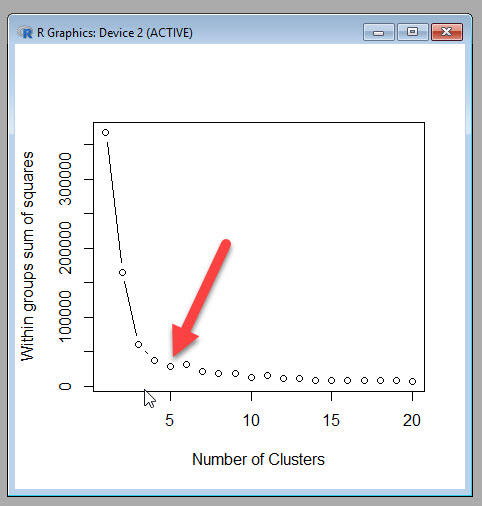Now Mr. Grogan’s plot a nice dramatic drop off, which is unfortunately not how most real world data I have seen works. I am going to chose 5 as my cut off point, because while the withinss does continue to decrease, it doesn’t seem to do so at a rate great enough to accept the added complexity of more clusters.

If you want to see how 5 clusters looks next to the three I had originally created, you can run the following code.

```myCluster <- kmeans(myData,5, nstart = 20)
myData\$cluster <- as.factor(myCluster\$cluster)
ggplot(myData, aes(TestA, TestB, color = cluster))
+ geom_point()```

5 Clusters3 Clusters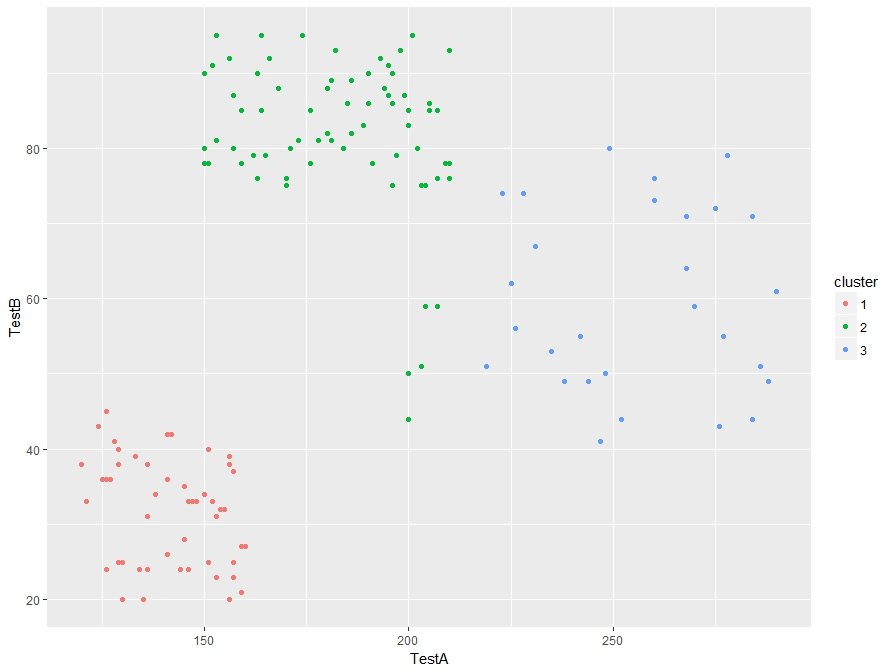I see some improvement in the 5 cluster model. So Michael Grogan’s trick for finding the number of clusters works.